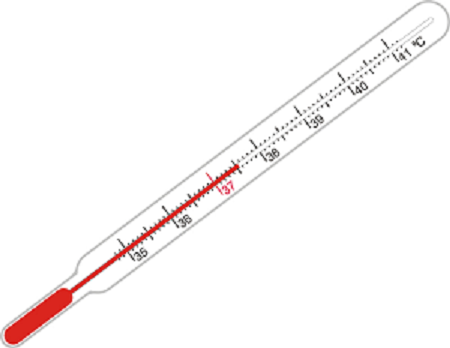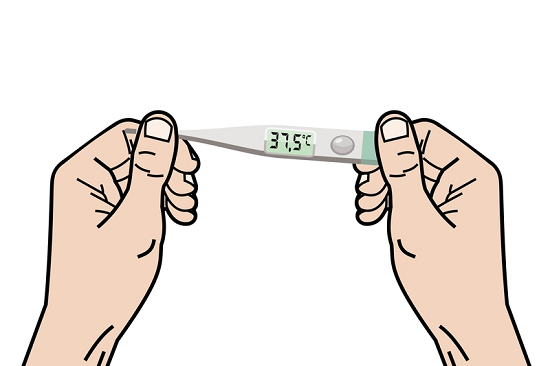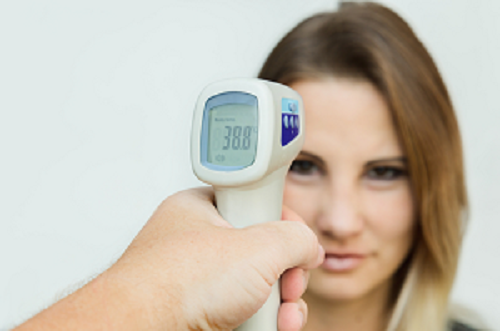# Difference Between Heat and Temperature

## Introduction

You must have used the terms temperature and heat synonymously at some point in your life. That is okay because, in the context of day-to-day usage, the two terms are quite similar. A greater amount of heat is characterized by a higher temperature. However, in the realm of Physics, heat, and temperature are closely related, but they aren’t congruent with each other. Indeed, while heat is a form of energy, the temperature is a measure of energy. Two items at the same temperature might not necessarily have the same amount of heat. Conversely, two items given the same amount of heat won’t necessarily have the same temperature.

In this article, we will elaborate on the concepts of heat and temperature and explain the differences between them.

## What is Heat?

Simply speaking, heat is a form of energy. In the realm of science, it is defined as energy that is in the process of transference into or out of a thermodynamic system. Now, as you will no doubt understand, energy can be transferred in a large number of ways. Thermodynamic work done is also energy transferred. So, is everything characterized as heat?

No, the only energy that is transferred via processes other than work done and matter transfer is known as heat. Examples of such processes can include conduction, radiation, friction, convection, etc.

## Units of Heat

Heat in the SI system is measured in Joules (J).

However, depending on the ease of use, one can also measure heat in other units like the calories. The amount of heat we would need to provide a gram of water to raise its temperature by 1° C, is defined as the calories.

Note that Calorie isn’t commonly used in Physics and engineering. Most of the time, scientists prefer the SI unit of Joules.

Let us discuss a few common misconceptions people hold about the heat.

### Heat and Cold are Different

As such, “cold” is nothing in the context of science. If we say that an object is cold, we are saying that it lacks heat. Cold is the absence of heat.

### Cold can Flow in or Out of an Object

As previously mentioned, cold is just the absence of heat. Thus, while cooling down a system, we are not pumping “cold” into it. Instead, we are removing heat from inside it.

### Temperature is a Measure of Heat

While we will soon discuss temperature in the following sections, you must understand that temperature does not measure heat. Instead, it is related to kinetic energy. Objects with the same amounts of heat might not have the same temperature.

## Main Sources of Heat Energy

Heat energy can be obtained from a large number of sources. Here are a few examples.

• The sun: The sun is the most distinctive source of heat for this planet. It radiates energy which arrives in the form of electromagnetic radiation (light) on Earth. This light is absorbed by the objects, causing them to gain heat energy.

• Energy from the Earth: The earth is teeming with heat energy. In a large number of places, one can find hot water springs, geysers, etc., whose heat can be used in a variety of scenarios like electricity generation. Energy obtained in this way is known as geothermal energy,

• Fuels: The most common, but also the most environmentally destructive way to generate heat is by burning fuels. While these fuels generate large amounts of heat, they also release various gases and pollute the environment thus, their use must be minimized.

## What Is Temperature?

Temperature is a measure of energy. While the temperature does express the degree of hotness, it is a measure of the average kinetic energy associated with the atoms or molecules of the system. The concept of temperature is used in almost every single field of science and is not limited to just Physics and engineering. Temperature affects a large number of physical processes. For instance, various chemical reactions occur only at certain temperatures.

## Different Scales of Temperature

Since temperature is a measure of heat and kinetic energy, there are multiple ways to define it. A particular scale of temperature describes how the temperature is measured and what one unit of the temperature corresponds to. Let us summarise the different scales of temperature:

• Celsius scale: The Celsius scale is named after a Swedish astronomer. Absolute zero and the triple point of a special type of water were used to define this scale. Absolute zero corresponds to the minimum possible theoretical temperature of 0 Kelvin and the triple point of water is 273.16 K.

• Thermodynamic scale or Kelvin scale: The thermodynamic scale is an absolute scale of temperature that is based entirely on the laws of thermodynamics. It was originally devised by Lord Kelvin using the efficiency of heat engines and the Carnot theorem.

• Fahrenheit Scale: The Fahrenheit scale originally used the freezing point of a special solution of brine as 0o F, and the average human body temperature at 96o F. Later, it was redefined using the Kelvin scale.

The Fahrenheit scale is commonly used in the United States of America now, while the international convention is to use the Kelvin scale.

## What is a Thermometer?

A device used to measure temperature is known as a thermometer. It usually consists of two important parts. The first is the temperature sensor, which can be digital or analog. The second is a display that converts the temperature measured into a numerical value on some scale, most commonly the Celsius or Fahrenheit scale.

The first thermometer is said to have been invented somewhere around the late 1500s. However, the first practical thermometer was designed in the early 18th century by Daniel Fahrenheit, who created the mercury in glass thermometer.

## Types of Thermometers

There are a large number of ways to classify thermometers into various types. Broadly, one can classify them into digital and analog, depending on how they sense and display the temperature reading. The most common types of thermometers are listed below:

• Mercury thermometer: A mercury thermometer has a small amount of mercury placed inside its bulb (the part that measures the temperature). Mercury expands upon heating and rises in the tube attached to the bulb. The amount by which it rises can be calibrated and the temperature reading listed on the tube.Fig:1 Clinical thermometer

• Digital thermometers: Digital thermometers have digital sensors and displays. The user places the bulb in their mouth or under the armpit for a fixed time interval and the output is displayed on a small screen to them.Fig:2 Digital thermometer

• Infrared thermometers: These are the most modern, accurate, and easy-to-use thermometers. They utilize the radiation emitted from the target body to sense its temperature and thus, can display the output instantaneously. They have the advantage of being non-contact instruments.Fig:3 Infrared thermometer

## Conclusion

Heat and temperature are sometimes used synonymously, but they are different concepts. Heat is a form of energy that is measured in Joules or Calories, while temperature is a measure of the degree of hotness. It is related to average kinetic energy and is measured in units like Celsius, Fahrenheit, etc. Major sources of heat include the sun, geothermal sources, and fossil fuels. The last one of these is dangerous to the environment and must be avoided. Temperature is measured via thermometers, which were first invented around the 1600s. They consist of a part to measure temperature (the bulb), and a part to display this temperature in certain units. Infrared thermometers are the most modern and accurate thermometers.

## FAQs

Q1. How do we convert between Fahrenheit and Celsius scales?

Ans. The Fahrenheit and Celsius scale have a linear relation. The following equation may be used:

$\mathrm{F=\frac{9}{5}C+32}$

Q2. What is the relation between the Celsius and Kelvin scale?

Ans. To convert from the Celsius to the Kelvin scale, add 273.15. For example, 32° C is equal to $\mathrm{32^{\circ}C+273.15=305.15K}$

Q3. Which temperature scale is most commonly used across the world?

Ans. The Celsius scale has the most practical usage by the common people. However, scientists use the Kelvin scale.

Q4. Is it possible to attain absolute zero?

Ans. So far, the notion of absolute zero is theoretical and we have been unable to reach it. However, close enough temperatures have been measured in laboratories.

Q5. Why do materials expand upon heating?

Ans. Heat is a measure of energy. Heated molecules vibrate more rapidly and with larger amplitudes, causing the material to expand.

Updated on: 11-Jan-2023

430 Views